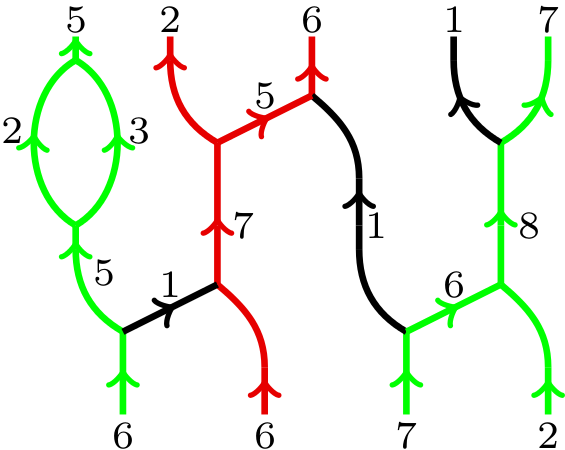## Data

• Title: Super $q$-Howe duality and web categories
• Authors: Daniel Tubbenhauer, Pedro Vaz and Paul Wedrich
• Status: Algebr. Geom. Topol. 17-6 (2017), 3703-3749. Last update: Tue, 21 Nov 2017 19:09:56 GMT
• ArXiv link: http://arxiv.org/abs/1504.05069
• ArXiv version = 0.99 published version
• LaTex Beamer presentation: Slides1, Slides2, Slides3, Slides4, Slides5, Slides6
• Poster: Poster

## Abstract

We use super $q$-Howe duality to provide diagrammatic presentations of an idempotented form of the Hecke algebra and of categories of $\mathfrak{gl}_N$-modules (and more generally $\mathfrak{gl}_{N|M}$-modules) whose objects are tensor generated by exterior and symmetric powers of the vector representations. As an application, we give a representation theoretic explanation and a diagrammatic version of a known symmetry of colored HOMFLY-PT polynomials.

## A few extra words

We discuss a machine that “takes dualities and produces diagrammatic presentations of the related representation theoretical categories”. Specifically, we feed the machine with super $q$-Howe duality between the superalgebra $\textbf{U}_q(\mathfrak{gl}(m|n))$ and $\textbf{U}_q(\mathfrak{gl}_N)$.
We construct diagrammatic presentations of an idempotented form of the Iwahori-Hecke algebra as well as of categories of $\textbf{U}_q(\mathfrak{gl}_N)$-modules by using the “green-red” web categories $\infty\text{-}\!\textbf{Web}_{\mathrm{gr}}$ and $N\text{-}\!\textbf{Web}_{\mathrm{gr}}$. Morphisms in these $\mathbb{C}_q$-linear categories are combinations of planar, upwards oriented, trivalent graphs with edges labeled by positive integers and colored black, green or red modulo local relations.
An example of a green-red web is:A very similar approach works for the corresponding categories of $\mathfrak{gl}_{N|M}$-modules as well as we show in an extra section.